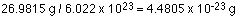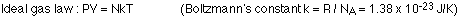## The gas laws

12-1-99

Sections 13.7 - 13.10

### A bit of chemistry

Let's delve into some concepts that you might associate with chemistry, but which are equally relevant to physics. The first is the idea of the mole, and Avogadro's number. A mole is a number like a dozen, just a lot bigger. A dozen means 12; a mole means 6.022 x 1023. That number, 6.022 x 1023, is known as Avogadro's number.

You can have a mole of eggs, just as you can have a dozen eggs, but a mole is more useful when it's applied to elements. A mole of aluminum and a mole of lead both have 6.022 x 1023 atoms. The mole of lead has more mass, though, because a mole of an element has a mass in grams equal to the atomic mass listed in the periodic table of elements. A mole of lead, then, has a mass of 207.2 g while a mole of aluminum has a mass of 26.9815 g.

A mole of an element has a mass conveniently measured in grams. A single atom, on the other hand, has a mass which is just a small fraction of a gram. A single atom of aluminum, for example, has a mass of:Note that this is the mass of an average atom of aluminum, accounting for different isotopes.

The mass of individual atoms are more conveniently measured in atomic mass units (u). 1 u = 1.66 x 10-24 g = 1.66 x 10-27 kg. For aluminum, then, which has an atomic mass of 26.9815, one mole has a mass of 26.9815 g and an average atom has a mass of 26.9815 u.

### The gas laws

Although we're now familiar with the ideal gas law, which relates the pressure, volume, and temperature of an ideal gas in one compact equation, it's useful to spend a few minutes on the history of gas laws. Three names in particular are associated with gas laws, those being Robert Boyle (1627 - 1691), Jacques Charles (1746-1823), and J.L. Gay-Lussac (1778-1850).

Boyle showed that for a fixed amount of gas at constant temperature, the pressure and volume are inversely proportional to one another. In other words:

Boyle's law : PV = constant.

In Charles' law, it is the pressure that is kept constant. Under this constraint, the volume is proportional to the temperature. This can be expressed as :

Charles' law : V1 / T1 = V2 / T2

When the volume is kept constant, it is the pressure of the gas that is proportional to temperature:

Gay-Lussac's law : P1 / T1 = P2 / T2

All of the above laws are combined in the ideal gas law. Before stating that, we should summarize what constitutes an ideal gas.

### An ideal gas

An ideal gas has a number of properties; real gases often exhibit behavior very close to ideal. The properties of an ideal gas are:

1. An ideal gas consists of a large number of identical molecules.
2. The volume occupied by the molecules themselves is negligible compared to the volume occupied by the gas.
3. The molecules obey Newton's laws of motion, and they move in random motion.
4. The molecules experience forces only during collisions; any collisions are completely elastic, and take a negligible amount of time.

### The ideal gas law

An ideal gas is an idealized model of real gases; real gases follow ideal gas behavior if their density is low enough that the gas molecules don't interact much, and when they do interact they undergo elastic collisions, with no loss of kinetic energy.

The behavior of an ideal gas, that is, the relationship of pressure (P), volume (V), and temperature (T), can be summarized in the ideal gas law:

Ideal gas law : PV = nRT

where n is the number of moles of gas, and R = 8.31 J / (mol K) is known as the universal gas constant.

An alternate way to express the ideal gas law is in terms of N, the number of molecules, rather than n, the number of moles. N is simply n multiplied by Avogadro's number, so the ideal gas law can be written as:We'll come back to the ideal gas law, but let's back up a little and get a feel for pressure on the molecular level. This gets us into the theory of the motion of gas molecules, known as the kinetic theory of gases.